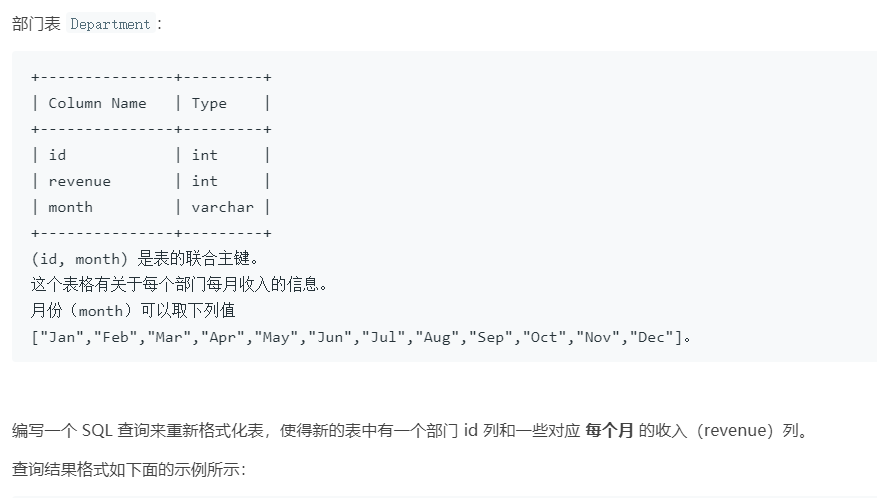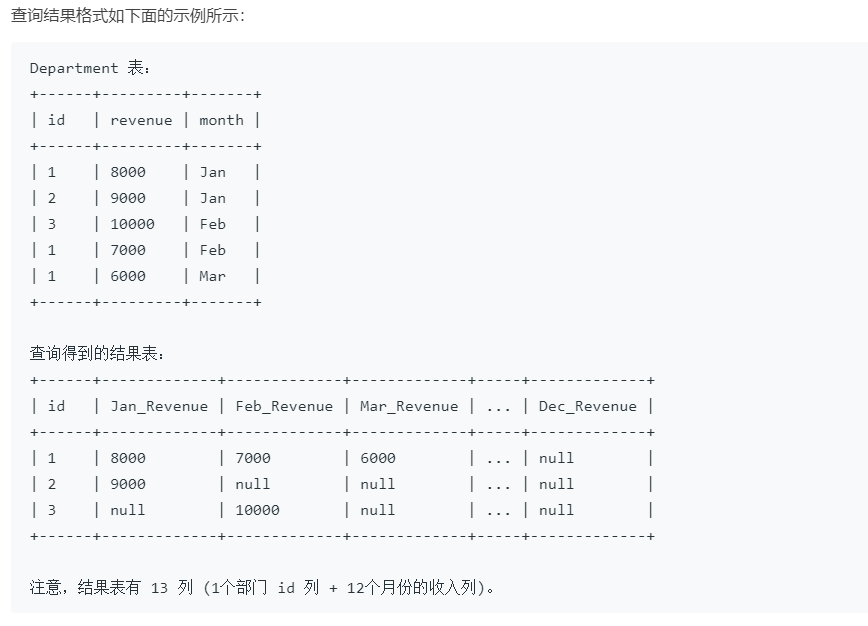# 1179. 重新格式化部门表

• 时间:

## 1179. 重新格式化部门表``````select
id
, sum(case `month` when 'Jan' then revenue else null end) as Jan_Revenue
, sum(case `month` when 'Feb' then revenue else null end) as Feb_Revenue
, sum(case `month` when 'Mar' then revenue else null end) as Mar_Revenue
, sum(case `month` when 'Apr' then revenue else null end) as Apr_Revenue
, sum(case `month` when 'May' then revenue else null end) as May_Revenue
, sum(case `month` when 'Jun' then revenue else null end) as Jun_Revenue
, sum(case `month` when 'Jul' then revenue else null end) as Jul_Revenue
, sum(case `month` when 'Aug' then revenue else null end) as Aug_Revenue
, sum(case `month` when 'Sep' then revenue else null end) as Sep_Revenue
, sum(case `month` when 'Oct' then revenue else null end) as Oct_Revenue
, sum(case `month` when 'Nov' then revenue else null end) as Nov_Revenue
, sum(case `month` when 'Dec' then revenue else null end) as Dec_Revenue
from Department group by id
``````

``````SELECT id,
SUM(CASE `month` WHEN 'Jan' THEN revenue END) Jan_Revenue,
SUM(CASE `month` WHEN 'Feb' THEN revenue END) Feb_Revenue,
SUM(CASE `month` WHEN 'Mar' THEN revenue END) Mar_Revenue,
SUM(CASE `month` WHEN 'Apr' THEN revenue END) Apr_Revenue,
SUM(CASE `month` WHEN 'May' THEN revenue END) May_Revenue,
SUM(CASE `month` WHEN 'Jun' THEN revenue END) Jun_Revenue,
SUM(CASE `month` WHEN 'Jul' THEN revenue END) Jul_Revenue,
SUM(CASE `month` WHEN 'Aug' THEN revenue END) Aug_Revenue,
SUM(CASE `month` WHEN 'Sep' THEN revenue END) Sep_Revenue,
SUM(CASE `month` WHEN 'Oct' THEN revenue END) Oct_Revenue,
SUM(CASE `month` WHEN 'Nov' THEN revenue END) Nov_Revenue,
SUM(CASE `month` WHEN 'Dec' THEN revenue END) Dec_Revenue
FROM Department
GROUP BY id;
``````